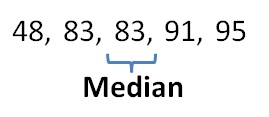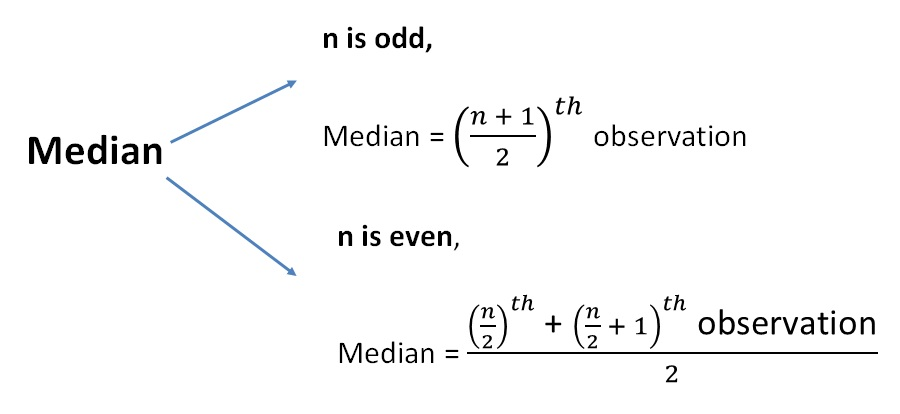Median

Chapter 3 Class 7 Data Handling
Concept wise

Median is the middle most value

Let’s take an example

Runs scored by Virat Kohli are 120, 24, 48, 64, 29, 64, 71, 3, 64, 29

Find Median

First, we arrange the numbers in ascending order

3, 24, 29, 48, 64, 64, 64, 71, 120

Here, Number of observations = 10

Since number of observations is even,

3, 24, 29, 48, 64, 64, 64, 71, 120

Median = ((10/2) th observation + (10/2  + 1) th   observation)/2

= (5 th  observation + 6 th   observation)/2

= (48 + 64)/2

= 112/2

= 56

So, median = 56

### Find median

First, we arrange the numbers in ascending orderMedian = Middle most observation = 83

We can also use the formula

Number of observations = 5

Since number of observation is odd

Median = ((n + 1)/2) th observation

= ((5 + 1)/2) th observation

= (6/2) th observation

= 3 rd observation

= 83

So, our formula isLearn in your speed, with individual attention - Teachoo Maths 1-on-1 Class

### Transcript

Marks scored by Satvik in his test are 48, 91, 83, 83, 95. Find median First, we arrange the numbers in ascending order Median = Middle most observation = 83 We can also use the formula Number of observations = 5 Since number of observation is odd Median = ((𝑛 + 1)/2)^𝑡ℎ observation = ((5 + 1)/2)^𝑡ℎ observation = (6/2)^𝑡ℎ observation = 3rd observation = 83 So, our formula is n is odd, Median = ((𝑛 + 1)/2)^𝑡ℎ observation n is even, Median = ((𝑛/2)^𝑡ℎ " + " ((𝑛 + 1)/2)^𝑡ℎ " observation" )/2